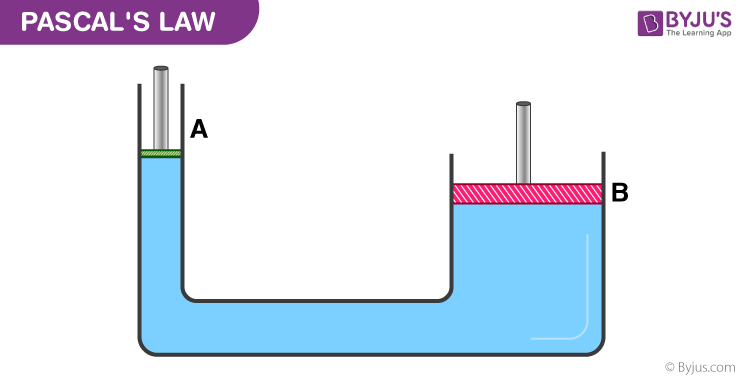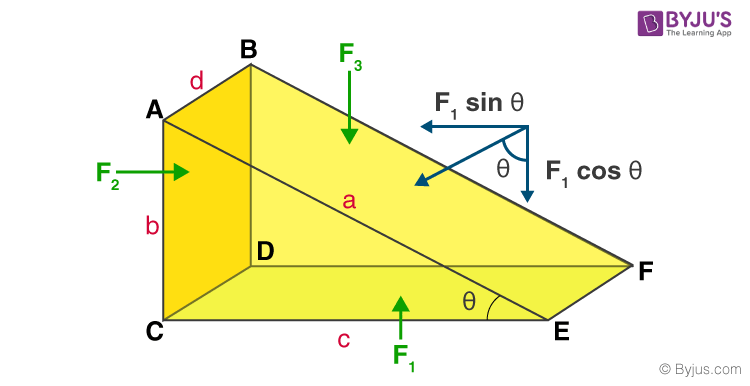Checkout JEE MAINS 2022 Question Paper Analysis : Checkout JEE MAINS 2022 Question Paper Analysis :

# Pascal's Law

Consider the image given below. The column is filled with water, and a piston has blocked the ends of each column A and B. If piston A is pressed, what do you think will happen to piston B? Piston B will rise. We have just applied Pascal’s law to our fluid pressure. Let us learn what Pascal’s law is.

## What is Pascal’s Law?

According to Pascal’s Law,

“The external static pressure applied on a confined liquid is distributed or transmitted evenly throughout the liquid in all directions”.

The static pressure acts at right angles to any surface in contact with the fluid. Pascal also found that the pressure at a point for a static fluid would be the same across all planes passing through that point in that fluid. Pascal’s law is also known as Pascal’s principle or principle of transmission of fluid-pressure. In 1653, Pascal law was stated by French mathematician Blaise Pascal.## Pascal Law Formula

Following is the formula of Pascal law:

 F = PA

Where F is the force applied, P is the pressure transmitted, and A is the cross-sectional area.

Related Articles:

## Example of Pascal’s Law

Let us understand the working principle of Pascal’s law with an example.

A pressure of 2000 Pa is transmitted throughout a liquid column due to a force being applied on a piston. If the piston has an area of 0.1 m2, what force is applied?

This can be calculated using Pascal’s Law formula.

F = PA

Here,

P = 2000 Pa = N/m2

A = 0.1 m2

Substituting values, we arrive at F = 200 N

## Applications of Pascal’s Law

• Hydraulic Lift: The image you saw at the beginning of this article is a simple line diagram of a hydraulic lift. This is the principle of the working of hydraulic lift. It works based on the principle of equal pressure transmission throughout a fluid (Pascal’s Law).
• The construction is such that a narrow cylinder (in this case A) is connected to a wider cylinder (in this case B). They are fitted with airtight pistons on either end. The inside of the cylinders is filled with fluid that cannot be compressed.
• Pressure applied at piston A is transmitted equally to piston B without diminishing the use of the fluid that cannot be compressed. Thus, piston B effectively serves as a platform to lift heavy objects like big machines or vehicles. A few more applications include a hydraulic jack and hydraulic press, and forced amplification is used in the braking system of most cars.

## Pascal’s Law Derivation

Consider an arbitrary right-angled prismatic triangle in the liquid of density rho. Since the prismatic element is very small, every point is considered to be at the same depth as the liquid surface. Therefore, T is also the same at all these points.

Consider an arbitrary right-angled prismatic triangle in the liquid of density rho. Since the prismatic element is very small, every point is considered to be at the same depth as the liquid surface. Therefore, the effect of gravity is also the same at all these points.Let ad, bd, and cd be the area of the faces ABFE, ABDC, and CDFE, respectively.

Let P1, P2, and P3 be the pressure on the faces ABFE, ABDC, and CDFE.

Pressure exerts a force which is normal to the surface. Let P1 exert force F1 on the surface ABFE, P2 exert force F2 on the surface ABDC, and P3 exert force F3 on the surface CDFE.

Therefore, Force F1, F2, and F3 is given as:
F1 = P1 × area of ABFE = P1 ad
F2 = P2 × area of ABDC = P2 bd
F3 = P3 × area of CDFE = P3 cd

Also,

$$\begin{array}{l}sin\theta =\frac{b}{a}\end{array}$$

$$\begin{array}{l}cos\theta =\frac{c}{a}\end{array}$$

The net force on the prism will be zero since the prism is in equilibrium.

F1 sin θ = F2
F1 cos θ = F3

$$\begin{array}{l}\frac{b}{a}\end{array}$$
= P2 bd (equ 1)
$$\begin{array}{l}\frac{c}{a}\end{array}$$
= P3 cd (equ 2)

From 1 and 2
P1 = P2 and P1 = P3

∴ P1= P2= P3

See the video below to learn about the variation of pressure in fluids.## Frequently Asked Questions – FAQs

### What is Pascal’s Law?

According to Pascal’s Law, “The external static pressure applied on a confined liquid is distributed or transmitted evenly throughout the liquid in all directions.”

### What is the application of Pascal’s Law?

Hydraulic lift work on the principle of Pascal’s Law.

### Does Pascal’s Law apply to gases?

Pascal’s Law applies to gases. Pascal’s principle is also known as the principle of transmission of fluid (which is water or gas) pressure.

### Who stated Pascal’s Law?

French mathematician Blaise Pascal stated the Pascal law in 1653.

### What is the principle of Pascal’s Law?

Pascal’s law says that pressure applied to an enclosed fluid will be transmitted without a change in magnitude to every point of the fluid and the walls of the container. The pressure at any point in the fluid is equal in all directions.

Stay tuned with BYJU’S to learn more interesting science topics with engaging videos!

Test your knowledge on Pascals Law And Its Application